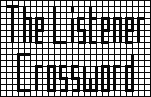## Listener Crossword 4112: Solution NotesBack to 2010 menu

## Girl by Xanthippe

### Puzzle explanation

One set of letters from the extra words in clues spelt “fold over two dashed lines; cut along thick lines”. Numbers in the grid indicated the dashed line positions. Folds should then have been made so that the northwest quadrant was TOPSIDE and the northeast quadrant LOWEST. Cutting through the thick lines visible on the northwest quadrant resulted in 16 cells becoming detached from the puzzle. These letters were to be arranged to give “a penny drop moment” and the grid submitted with six holes. Letters from extra words not used in the instruction gave a definition for a penny, ie, “formerly a two hundred and fortieth of one pound”.

### Clue explanations

Conventions: * = anagram, < = reversal

 Extra Answer Explanation ACROSS (F)un(F)air TISRI (ten)T IS RI(otous) (O)ct(O)genarian AVAL AVAIL − I (R)ec(L)use OLEA O + LEA(n) (D)al(M)atian MORE MOROSE − OS (E)xp(O)sed PLACER PR around (L + ACE) (V)ag(R)ant BREW HEBREW − HE (L)ak(E)s SOLE 2 meanings (Y)ea(R) APOGEE (O + PA) < + GEE (T)en(A)nts’ IMARI I + MAR + I’ (W)ai(T)ers CRESS R in CHESS − (t)H(e) (O)ut(W)orker DEN DEN(y) (O)we(D) DROPLET D(ocke)T around P in ROLE (A)rc(H)itect ENTERTAINS (INAN(e) STREET) * (S)co(U)ts’ BEER GARDEN E in (DRAB GREEN) * (N)ot(H)ing YORE YORK with E for K (D)ev(E)loped ECOLOGIC E + GO < in COLIC (R)ea(D) AD REM PADRE − P + M (E)xp(L)oded SACK 2 meanings (D)iv(I)ded KAMPONG KG around (A + MP + ON) (A)ge(N)t IRAN AIR * + N (E)ve(N)ly TENON NONET < (S)tu(D)io GLOSSIER GLOSSER around I DOWN (F)ar(C)ical TOPSIDE (O(f) + P + SIDE) after T(itle) (O)cc(U)pant ILL-OMENED I + (DEN + E + MOLL) < (R)es(T)ored SEALANT SET around (AL + A + (pa)N(els)) (A)us(T)ralian RACER R(eddish) + ACER (I)ta(L)ian DORP DOUR − U + P (E)xp(O)sed ARBOR (BARROW − W) * (T)hi(N) VERGE 2 meanings (G)ot(H)ic LOWEST LOWESTOFT − OFT (T)al(O)ns GEESE SEE < in G(abl)E (F)as(H)ionable COTANGENTS (AGENT around N after COST) with S moved to the end (O)rd(I)nary LIDOCAINE L(ocal) I(njected) + MAINE with DOC for M(olar) (C)ha(N)ging ERE homophone of AIR (K)it(E) SNEAKING SNAKING around (lin)E (P)ul(L)ed BREAK-IN BEAK around (d)R(ivers) + IN (O)bl(I)quely GNOMONS MONG < + ON + S(undial) (N)at(U)re FORMAL FOR + MAL (E)co(N)omic RECTOR REC + ROT < (S)tu(D)ent DARG GRAD <
Back to 2010 menu